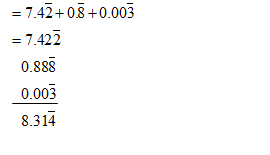• This is an assessment test.
• These tests focus on the basics of Maths and are meant to indicate your preparation level for the subject.
• Kindly take the tests in this series with a pre-defined schedule.

## Basic Maths: Test 45

Congratulations - you have completed Basic Maths: Test 45.

You scored %%SCORE%% out of %%TOTAL%%.

Your performance has been rated as %%RATING%%

 Question 1
Which of the following is greater than $\left( 1-\frac{1}{100} \right)\,\left( 1-\frac{1}{101} \right)\,\left( 1-\frac{1}{102} \right)\,......\left( 1-\frac{1}{200} \right)$ :
 A 0.2 B 0.3 C 0.4 D 0.5
Question 1 Explanation:
\begin{align} & \left( 1-\frac{1}{100} \right)\,\left( 1-\frac{1}{101} \right)\,\left( 1-\frac{1}{102} \right)\,......\left( 1-\frac{1}{200} \right) \\ & =\frac{99}{100}\times \frac{100}{101}\times \frac{101}{102}\times .....\frac{199}{200}=\frac{99}{200} \\ \end{align}
Is less than 0.5
 Question 2
${{\left( \sqrt{3}+\frac{1}{\sqrt{3}} \right)}^{2}}$ is equal to:
 A $2\frac{1}{3}$ B $3\frac{1}{3}$ C $4\frac{1}{3}$ D $5\frac{1}{3}$
Question 2 Explanation:
\begin{align} & {{\left( \sqrt{3}+\frac{1}{\sqrt{3}} \right)}^{2}} \\ & =3+\frac{1}{3}+2\times \sqrt{3}\times \frac{1}{\sqrt{3}}=5\frac{1}{3} \\ \end{align}
 Question 3
The value of ${{\left( .89 \right)}^{3}}+{{\left( 0.11 \right)}^{3}}+3\times .89\times .11-1$ is:
 A 1.98 B 1.09 C 1 D 0
Question 3 Explanation:
Expression
\begin{align} & {{\left( .89 \right)}^{3}}+{{\left( 0.11 \right)}^{3}}+3\times .89\times .11-1 \\ & ={{\left( .89 \right)}^{3}}+{{\left( 0.11 \right)}^{3}}+3\times .89\times .11\left( 0.89+0.11 \right)-1 \\ & ={{\left( 0.89+0.11 \right)}^{3}}-1=1-1=0 \\ \end{align}
 Question 4
$7.4\overline{2}+0.\overline{8}+0.00\overline{3}$ is equal to
 A $8.\overline{313}$ B $8.3\overline{13}$ C $8.31\overline{3}$ D $8.3\overline{13}$
Question 4 Explanation:Question 5
The value of $\frac{4}{5}\times \frac{5}{\frac{7}{9}\div \frac{5}{3}\,\,of\,\,1\frac{6}{15}}$ is
 A 2.5 B 12 C 10 D 5
Question 5 Explanation:
Expression
\begin{align} & =\frac{4}{5}\times \frac{5}{\frac{7}{9}\div \frac{5}{3}\times \frac{21}{15}} \\ & =\frac{4}{5}\times 15=12 \\ \end{align}
Once you are finished, click the button below. Any items you have not completed will be marked incorrect.
There are 5 questions to complete.
 ← List →
Shaded items are complete.
 1 2 3 4 5 End